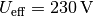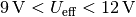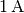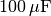# A diode can convert alternating current into direct current

## Rectifier and inverter¶

An AC voltage with an effective voltage of is applied to standard household socketsat. This voltage can easily be adjusted to the desired voltage value with built-in or external transformers ("power supply units").

However, many electronic components (for example electrolytic capacitors, LEDs, diodes and transistors) are not designed for operation with alternating voltage or alternating current. To operate circuits with such components, the alternating voltage must be converted into a correspondingly large direct voltage. This is done with so-called rectifiers.

Conversely, a direct voltage can also be converted into an alternating voltage. This is necessary, for example, in the radio and remote control area in order to control loudspeakers or light barriers with certain voltage frequencies. Another application arises in the photovoltaic area, where one often wants to feed the direct voltage provided by solar cells into the general power grid. In general, such circuits, which convert a direct voltage into a correspondingly large alternating voltage, are called inverters.

### Rectifier circuits¶

Rectifiers convert AC voltage into DC voltage. This requires components that only allow the current to pass in one direction and block it in the other. In the past, electron tubes were used for this purpose, but now semiconductor diodes are used almost exclusively.

For safe experimentation, the following circuits use an alternating voltage of approximatelyas provided by laboratory power supplies. 

### The half-wave rectifier¶

The easiest way to implement a rectifier is through the following circuit:

The circuit works as follows:

• If there is a positive voltage at the upper pole of the AC voltage source, the diode conducts. The capacitor charges up completely, at the same time current flows through the load resistor.
• If there is a positive voltage at the lower pole of the AC voltage source, the diode blocks. The capacitor discharges and thus briefly maintains the current flow through the load resistor.

If the load resistance is very high, only a very low current strength can occur. As a result, the capacitor only discharges slowly and can (largely) maintain the nominal voltage until the next polarity reversal. In practice, the load resistance is limited so that the voltage applied to the load resistance can drop sharply, possibly even to zero, in the meantime.

### Full-wave rectifier¶

By using four diodes - unlike a half-wave rectifier - both polarities of the power source, i.e. both half-arcs of the sinusoidal alternating voltage, can be used. The basic circuit diagram looks like this:

The circuit works as follows:

• If there is a positive voltage at the upper pole of the AC voltage source, in the current flow diagram of a two-way rectifier (left picture), current flows through the upper left diode to the capacitor or load resistor and back via the lower right diode to the lower pole of the AC voltage source.
• If there is a positive voltage at the lower pole of the AC voltage source, in the current flow diagram of a two-way rectifier (right picture), current flows through the upper right diode to the capacitor or load resistor and back via the lower left diode to the upper pole of the AC voltage source.

The output voltage is a pulsating DC voltage which, like a half-wave rectifier, is more or less smoothed by the capacitor.

Two-way rectifiers are often used in power supplies for small electronic devices. The special arrangement of the four diodes, also known as the “Grätz circuit”, is also available as a finished component (“bridge rectifier”).

As can be seen in the following figure, the amount of the DC voltage provided by a bridge rectifier is not constant over time; rather, a “pulsation” of the voltage can be clearly seen. In order to compensate for the "voltage dips", a capacitor can be connected in parallel to the actual consumer circuit.

The capacitor charges while the applied voltage is high and in turn maintains that value while the applied voltage is low. As a rule of thumb, a bridge rectifier should be behind eachat output current strength aboutto be installed on capacitor capacitance.

### Inverter circuits¶

Inverters convert direct voltage into alternating voltage. Pole changers are used for this purpose, which alternately connect the poles of the input DC voltage to the outputs (for example sockets) at a certain frequency.

In the simplest case, the pole changer is a changeover switch that is operated manually or electronically with the aid of a relay. The frequency of the alternating voltage corresponds to the switching frequency of the pole changer.

... to be continued ...

Annotation: